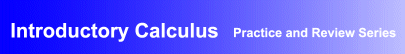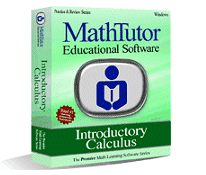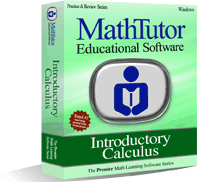Award-Winning

# Calculus Review and Practice SoftwareDESCRIPTION
INTRODUCTORY CALCULUS is a widely praised drill and practice software package designed to help students build problem solving skills in first year calculus.
Through interactive, step by step explanations, INTRODUCTORY CALCULUS helps students determine what a problem is asking, and the steps that are required to carry out a solution.
Problems are generated using randomization algorithms that ensure problems are stimulating and appropriate for a student's skill level.
Problem solving becomes a vehicle for reinforcement of students' understanding of the concepts and principles of calculus.
Feedback and recordkeeping allow students to see the progress they have made, and topic areas where additional practice is recommended.
• Curriculum-based first year calculus drill and practice software developed by math educators
• Interactive, stimulating calculus problem solving exercises that are ideal for self-study, and as a supplement to classroom instruction
• Proven effectiveness in boosting students' understanding of concepts and proficiency in problem solvingTOPICS COVERED
Review of Algebra
• Products and Factors
• Rules of Exponents
• Exponents and Logarithms
• Word Problems: Test Scores
• Word Problems: Gas Mileage
Functions
• Evaluating Functions
• The Coordinate System
• Graphs of Straight Lines
• Graphs of Conics
• Graphs of Logs and Exponents
• Asymptotic Curves
• Delta Notation
• Average Rates of Change
Differential Calculus
• Sequences
• Limits of Sequences
• Function Properties I
• Differentiation I
• Differentiation II
• Maxima and Minima
• Word Problems: Brooms
• Implicit Differentiation
• L'Hospital's Rule
• Differentiable Functions
• Areas and Perimeters
Integral Calculus
• Indefinite Integrals I
• Indefinite Integrals II
• Definite Integrals
• Advanced Integration Techniques
• Bounded Area: Curves
• Bounded Area: Straight Lines
• Solids of Revolution